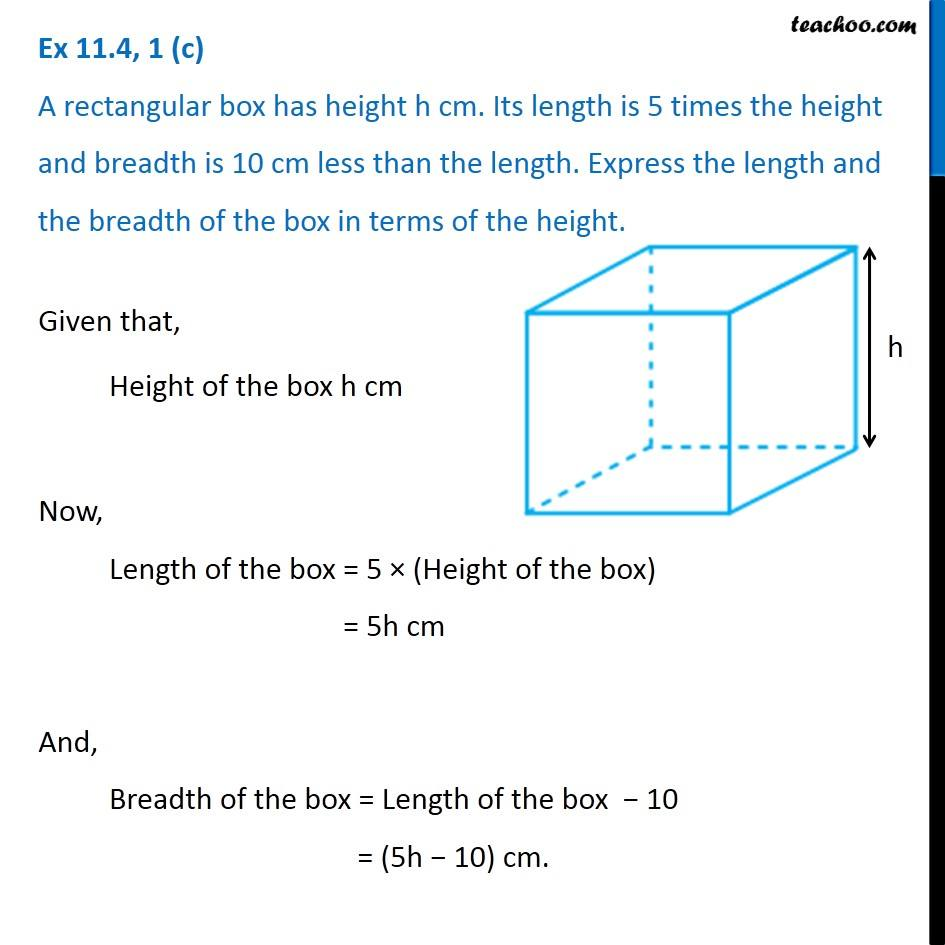1. Chapter 11 Class 6 Algebra
2. Concept wise
3. Statements to expressions

Transcript

Ex 11.4, 1 (c) A rectangular box has height h cm. Its length is 5 times the height and breadth is 10 cm less than the length. Express the length and the breadth of the box in terms of the height. Given that, Height of the box h cm Now, Length of the box = 5 × (Height of the box) = 5h cm And, Breadth of the box = Length of the box − 10 = (5h − 10) cm.